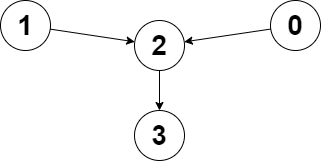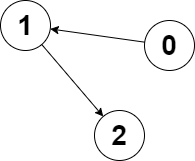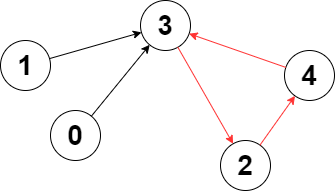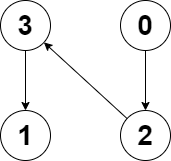# weekly-contest-304

## A

### Statement

• 选出一个正整数 `x``x` 需要小于或等于 `nums`最小非零 元素。
• `nums` 中的每个正整数都减去 `x`

``````输入：nums = [1,5,0,3,5]

``````输入：nums = 

``````

• `1 <= nums.length <= 100`
• `0 <= nums[i] <= 100`

You are given a non-negative integer array `nums`. In one operation, you must:

• Choose a positive integer `x` such that `x` is less than or equal to the smallest non-zero element in `nums`.
• Subtract `x` from every positive element in `nums`.

Return the minimum number of operations to make every element in `nums` equal to `0`.

Example 1:

``````Input: nums = [1,5,0,3,5]
Output: 3
Explanation:
In the first operation, choose x = 1. Now, nums = [0,4,0,2,4].
In the second operation, choose x = 2. Now, nums = [0,2,0,0,2].
In the third operation, choose x = 2. Now, nums = [0,0,0,0,0].
``````

Example 2:

``````Input: nums = 
Output: 0
Explanation: Each element in nums is already 0 so no operations are needed.
``````

Constraints:

• `1 <= nums.length <= 100`
• `0 <= nums[i] <= 100`

### Solution

``````#include <bits/stdc++.h>
#include <ext/pb_ds/assoc_container.hpp>
#include <ext/pb_ds/tree_policy.hpp>

#define endl "\n"
#define fi first
#define se second
#define all(x) begin(x), end(x)
#define rall rbegin(a), rend(a)
#define bitcnt(x) (__builtin_popcountll(x))
#define complete_unique(a) a.erase(unique(begin(a), end(a)), end(a))
#define mst(x, a) memset(x, a, sizeof(x))
#define MP make_pair

using ll = long long;
using ull = unsigned long long;
using db = double;
using ld = long double;
using VLL = std::vector<ll>;
using VI = std::vector<int>;
using PII = std::pair<int, int>;
using PLL = std::pair<ll, ll>;

using namespace __gnu_pbds;
using namespace std;
template <typename T>
using ordered_set = tree<T, null_type, less<T>, rb_tree_tag, tree_order_statistics_node_update>;

template <typename T, typename S>
inline bool chmax(T &a, const S &b) {
return a < b ? a = b, 1 : 0;
}

template <typename T, typename S>
inline bool chmin(T &a, const S &b) {
return a > b ? a = b, 1 : 0;
}

#ifdef LOCAL
#include <debug.hpp>
#else
#define dbg(...)
#endif

class Solution {
public:
int minimumOperations(vector<int> &nums) {
sort(all(nums));
nums.erase(unique(all(nums)), end(nums));
int res = 0;
for (const auto &a : nums) {
res += (a > 0);
}

return res;
}
};

#ifdef LOCAL

int main() {
return 0;
}

#endif
``````

## B

### Statement

• `i` 个分组中的学生总成绩 小于`(i + 1)` 个分组中的学生总成绩，对所有组均成立（除了最后一组）。
• `i` 个分组中的学生总数 小于`(i + 1)` 个分组中的学生总数，对所有组均成立（除了最后一组）。

``````输入：grades = [10,6,12,7,3,5]

- 第 1 个分组的学生成绩为 grades =  ，总成绩：12 ，学生数：1
- 第 2 个分组的学生成绩为 grades = [6,7] ，总成绩：6 + 7 = 13 ，学生数：2
- 第 3 个分组的学生成绩为 grades = [10,3,5] ，总成绩：10 + 3 + 5 = 18 ，学生数：3

``````

``````输入：grades = [8,8]

``````

• `1 <= grades.length <= 105`
• `1 <= grades[i] <= 105`

You are given a positive integer array `grades` which represents the grades of students in a university. You would like to enter all these students into a competition in ordered non-empty groups, such that the ordering meets the following conditions:

• The sum of the grades of students in the `ith` group is less than the sum of the grades of students in the `(i + 1)th` group, for all groups (except the last).
• The total number of students in the `ith` group is less than the total number of students in the `(i + 1)th` group, for all groups (except the last).

Return the maximum number of groups that can be formed.

Example 1:

``````Input: grades = [10,6,12,7,3,5]
Output: 3
Explanation: The following is a possible way to form 3 groups of students:
- 1st group has the students with grades = . Sum of grades: 12. Student count: 1
- 2nd group has the students with grades = [6,7]. Sum of grades: 6 + 7 = 13. Student count: 2
- 3rd group has the students with grades = [10,3,5]. Sum of grades: 10 + 3 + 5 = 18. Student count: 3
It can be shown that it is not possible to form more than 3 groups.
``````

Example 2:

``````Input: grades = [8,8]
Output: 1
Explanation: We can only form 1 group, since forming 2 groups would lead to an equal number of students in both groups.
``````

Constraints:

• `1 <= grades.length <= 105`
• `1 <= grades[i] <= 105`

### Solution

``````#include <bits/stdc++.h>
#include <ext/pb_ds/assoc_container.hpp>
#include <ext/pb_ds/tree_policy.hpp>
#include <vector>

#define endl "\n"
#define fi first
#define se second
#define all(x) begin(x), end(x)
#define rall rbegin(a), rend(a)
#define bitcnt(x) (__builtin_popcountll(x))
#define complete_unique(a) a.erase(unique(begin(a), end(a)), end(a))
#define mst(x, a) memset(x, a, sizeof(x))
#define MP make_pair

using ll = long long;
using ull = unsigned long long;
using db = double;
using ld = long double;
using VLL = std::vector<ll>;
using VI = std::vector<int>;
using PII = std::pair<int, int>;
using PLL = std::pair<ll, ll>;

using namespace __gnu_pbds;
using namespace std;
template <typename T>
using ordered_set = tree<T, null_type, less<T>, rb_tree_tag, tree_order_statistics_node_update>;

template <typename T, typename S>
inline bool chmax(T &a, const S &b) {
return a < b ? a = b, 1 : 0;
}

template <typename T, typename S>
inline bool chmin(T &a, const S &b) {
return a > b ? a = b, 1 : 0;
}

#ifdef LOCAL
#include <debug.hpp>
#else
#define dbg(...)
#endif

class Solution {
public:
int maximumGroups(vector<int> &g) {
sort(all(g));

auto v = vector<pair<ll, int>>();

for (int i = 0; i < int(g.size()); ++i) {
if (int(v.size()) < 2) {
v.emplace_back(g[i], 1);
continue;
}

if (int(v.size()) > 1) {
if (v.end()[-1].fi > v.end()[-2].fi && v.end()[-1].se > v.end()[-2].se) {
v.emplace_back(g[i], 1);
continue;
}
}

v.back().fi += g[i];
++v.back().se;
}

while (v.size() >= 2) {
if (v.end()[-1].fi > v.end()[-2].fi && v.end()[-1].se > v.end()[-2].se) {
break;
}

auto bk = v.back();
v.pop_back();

v.back().fi += bk.fi;
v.back().se += bk.se;
}

return int(v.size());
}
};

#ifdef LOCAL

int main() {
return 0;
}

#endif
``````

## C

### Statement``````输入：edges = [2,2,3,-1], node1 = 0, node2 = 1

````````````输入：edges = [1,2,-1], node1 = 0, node2 = 2

``````

• `n == edges.length`
• `2 <= n <= 105`
• `-1 <= edges[i] < n`
• `edges[i] != i`
• `0 <= node1, node2 < n`

You are given a directed graph of `n` nodes numbered from `0` to `n - 1`, where each node has at most one outgoing edge.

The graph is represented with a given 0-indexed array `edges` of size `n`, indicating that there is a directed edge from node `i` to node `edges[i]`. If there is no outgoing edge from `i`, then `edges[i] == -1`.

You are also given two integers `node1` and `node2`.

Return the index of the node that can be reached from both `node1` and `node2`, such that the maximum between the distance from `node1` to that node, and from `node2` to that node is minimized. If there are multiple answers, return the node with the smallest index, and if no possible answer exists, return `-1`.

Note that `edges` may contain cycles.

Example 1:``````Input: edges = [2,2,3,-1], node1 = 0, node2 = 1
Output: 2
Explanation: The distance from node 0 to node 2 is 1, and the distance from node 1 to node 2 is 1.
The maximum of those two distances is 1. It can be proven that we cannot get a node with a smaller maximum distance than 1, so we return node 2.
``````

Example 2:``````Input: edges = [1,2,-1], node1 = 0, node2 = 2
Output: 2
Explanation: The distance from node 0 to node 2 is 2, and the distance from node 2 to itself is 0.
The maximum of those two distances is 2. It can be proven that we cannot get a node with a smaller maximum distance than 2, so we return node 2.
``````

Constraints:

• `n == edges.length`
• `2 <= n <= 105`
• `-1 <= edges[i] < n`
• `edges[i] != i`
• `0 <= node1, node2 < n`

### Solution

``````#include <bits/stdc++.h>
#include <ext/pb_ds/assoc_container.hpp>
#include <ext/pb_ds/tree_policy.hpp>
#include <queue>
#include <vector>

#define endl "\n"
#define fi first
#define se second
#define all(x) begin(x), end(x)
#define rall rbegin(a), rend(a)
#define bitcnt(x) (__builtin_popcountll(x))
#define complete_unique(a) a.erase(unique(begin(a), end(a)), end(a))
#define mst(x, a) memset(x, a, sizeof(x))
#define MP make_pair

using ll = long long;
using ull = unsigned long long;
using db = double;
using ld = long double;
using VLL = std::vector<ll>;
using VI = std::vector<int>;
using PII = std::pair<int, int>;
using PLL = std::pair<ll, ll>;

using namespace __gnu_pbds;
using namespace std;
template <typename T>
using ordered_set = tree<T, null_type, less<T>, rb_tree_tag, tree_order_statistics_node_update>;

template <typename T, typename S>
inline bool chmax(T &a, const S &b) {
return a < b ? a = b, 1 : 0;
}

template <typename T, typename S>
inline bool chmin(T &a, const S &b) {
return a > b ? a = b, 1 : 0;
}

#ifdef LOCAL
#include <debug.hpp>
#else
#define dbg(...)
#endif

constexpr int INF = 0x3f3f3f3f;

class Solution {
public:
int closestMeetingNode(vector<int> &e, int node1, int node2) {
int n = int(e.size());
auto bfs = [&n, &e](int st) {
auto dis = vector<int>(n, INF);
dis[st] = 0;

queue<int> q;
q.push(st);

while (!q.empty()) {
int u = q.front();
q.pop();

int nx = e[u];
if (nx == -1) {
continue;
}

if (dis[nx] <= dis[u] + 1) {
continue;
}

q.push(nx);
dis[nx] = dis[u] + 1;
}

return dis;
};

auto dis1 = bfs(node1);
auto dis2 = bfs(node2);

int dis = INF;
int res = -1;

for (int i = 0; i < n; ++i) {
if (dis1[i] == INF || dis2[i] == INF) {
continue;
}

if (chmin(dis, max(dis1[i], dis2[i]))) {
res = i;
}
}

return res;
}
};

#ifdef LOCAL

int main() {
return 0;
}

#endif
``````

## D

### Statement``````输入：edges = [3,3,4,2,3]

````````````输入：edges = [2,-1,3,1]

``````

• `n == edges.length`
• `2 <= n <= 105`
• `-1 <= edges[i] < n`
• `edges[i] != i`

You are given a directed graph of `n` nodes numbered from `0` to `n - 1`, where each node has at most one outgoing edge.

The graph is represented with a given 0-indexed array `edges` of size `n`, indicating that there is a directed edge from node `i` to node `edges[i]`. If there is no outgoing edge from node `i`, then `edges[i] == -1`.

Return the length of the longest cycle in the graph. If no cycle exists, return `-1`.

A cycle is a path that starts and ends at the same node.

Example 1:``````Input: edges = [3,3,4,2,3]
Output: 3
Explanation: The longest cycle in the graph is the cycle: 2 -> 4 -> 3 -> 2.
The length of this cycle is 3, so 3 is returned.
``````

Example 2:``````Input: edges = [2,-1,3,1]
Output: -1
Explanation: There are no cycles in this graph.
``````

Constraints:

• `n == edges.length`
• `2 <= n <= 105`
• `-1 <= edges[i] < n`
• `edges[i] != i`

### Solution

``````#include <bits/stdc++.h>
#include <ext/pb_ds/assoc_container.hpp>
#include <ext/pb_ds/tree_policy.hpp>
#include <vector>

#define endl "\n"
#define fi first
#define se second
#define all(x) begin(x), end(x)
#define rall rbegin(a), rend(a)
#define bitcnt(x) (__builtin_popcountll(x))
#define complete_unique(a) a.erase(unique(begin(a), end(a)), end(a))
#define mst(x, a) memset(x, a, sizeof(x))
#define MP make_pair

using ll = long long;
using ull = unsigned long long;
using db = double;
using ld = long double;
using VLL = std::vector<ll>;
using VI = std::vector<int>;
using PII = std::pair<int, int>;
using PLL = std::pair<ll, ll>;

using namespace __gnu_pbds;
using namespace std;
template <typename T>
using ordered_set = tree<T, null_type, less<T>, rb_tree_tag, tree_order_statistics_node_update>;

template <typename T, typename S>
inline bool chmax(T &a, const S &b) {
return a < b ? a = b, 1 : 0;
}

template <typename T, typename S>
inline bool chmin(T &a, const S &b) {
return a > b ? a = b, 1 : 0;
}

#ifdef LOCAL
#include <debug.hpp>
#else
#define dbg(...)
#endif

class Solution {
public:
int longestCycle(vector<int> &e) {
int n = int(e.size());

auto vis = vector<int>(n, 0);
auto in_sta = vector<int>(n, 0);
int sta_ix = 0;
int res = -1;

auto dfs = [&vis, &in_sta, &sta_ix, &e, &res](auto &&dfs, int u) -> void {
vis[u] = 1;
in_sta[u] = ++sta_ix;

int nx = e[u];

if (nx != -1) {
if (in_sta[nx]) {
int _res = sta_ix - in_sta[nx] + 1;
res = max(res, _res);
}

if (!vis[nx]) {
dfs(dfs, nx);
}
}

in_sta[u] = 0;
--sta_ix;
};

for (int i = 0; i < n; i++) {
if (!vis[i]) {
dfs(dfs, i);
}
}

return res;
}
};

#ifdef LOCAL

int main() {
return 0;
}

#endif
``````